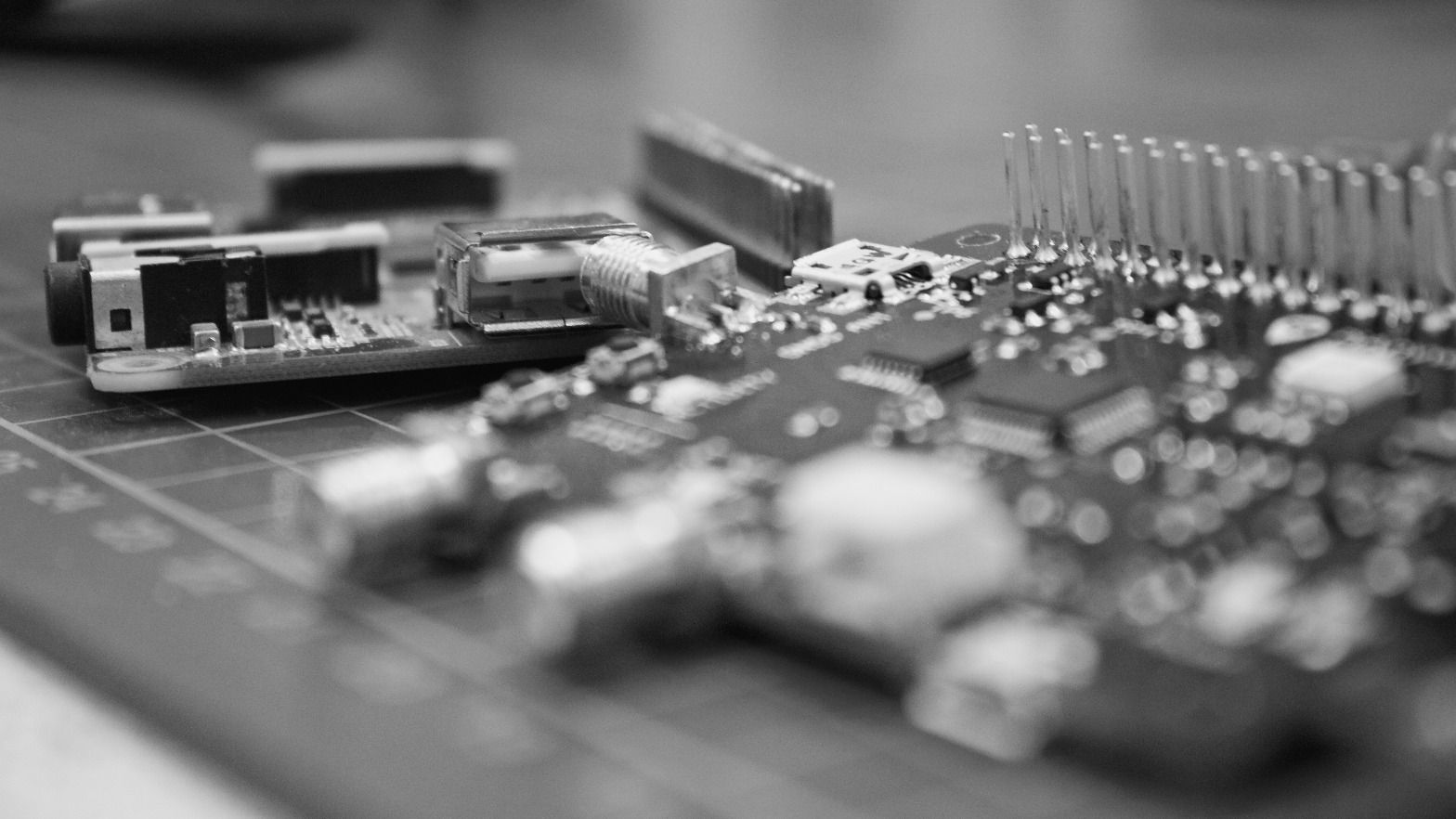# Let Us C SolutionsChapter 15: Operations On BitsLet Us C Solutions chapter 15, Operations on Bits. This chapter is about operations being done on the binary data.

A computer only understands binary language, that is 0s and 1s. Each 0 and 1 are stored in a memory unit called a bit. So here in this chapter, we will do the operation in bits. Some of them are AND, OR, XOR, etc.

## Sections

© 2021 Garbage Value